# aesara.tensor.random.multinomial#

aesara.tensor.random.multinomial = <aesara.tensor.random.basic.MultinomialRV object>[source]#

A multinomial discrete random variable.

The probability mass function of multinomial in terms of the number of experiments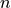and the probabilities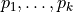of the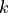different possible outcomes is: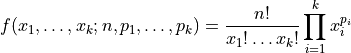where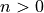and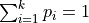.

Notes

The length of the support dimension is determined by the last dimension in the second parameter (i.e. the probabilities vector).